Case Based Questions Test: Triangles - 1

# Case Based Questions Test: Triangles - 1 - Class 10

Test Description

## 15 Questions MCQ Test Mathematics (Maths) Class 10 - Case Based Questions Test: Triangles - 1

Case Based Questions Test: Triangles - 1 for Class 10 2023 is part of Mathematics (Maths) Class 10 preparation. The Case Based Questions Test: Triangles - 1 questions and answers have been prepared according to the Class 10 exam syllabus.The Case Based Questions Test: Triangles - 1 MCQs are made for Class 10 2023 Exam. Find important definitions, questions, notes, meanings, examples, exercises, MCQs and online tests for Case Based Questions Test: Triangles - 1 below.
Solutions of Case Based Questions Test: Triangles - 1 questions in English are available as part of our Mathematics (Maths) Class 10 for Class 10 & Case Based Questions Test: Triangles - 1 solutions in Hindi for Mathematics (Maths) Class 10 course. Download more important topics, notes, lectures and mock test series for Class 10 Exam by signing up for free. Attempt Case Based Questions Test: Triangles - 1 | 15 questions in 30 minutes | Mock test for Class 10 preparation | Free important questions MCQ to study Mathematics (Maths) Class 10 for Class 10 Exam | Download free PDF with solutions
 1 Crore+ students have signed up on EduRev. Have you?
Case Based Questions Test: Triangles - 1 - Question 1

### Direction: Read the following text and answer the below questions: SCALE FACTOR A scale drawing of an object is the same shape at the object but a different size. The scale of a drawing is a comparison of the length used on a drawing to the length it represents. The scale is written as a ratio. The ratio of two corresponding sides in similar figures is called the scale factor Scale factor = length in image / corresponding length in objectIf one shape can become another using revising, then the shapes are similar. Hence, two shapes are similar when one can become the other after a resize, flip, slide or turn. In the photograph below showing the side view of a train engine. Scale factor is 1:200 This means that a length of 1 cm on the photograph above corresponds to a length of 200 cm or 2 m, of the actual engine. The scale can also be written as the ratio of two lengths. The length of AB in the given figure: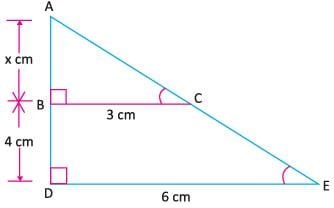Detailed Solution for Case Based Questions Test: Triangles - 1 - Question 1

Since, ΔABC and ΔADE are similar, then their ratio of corresponding sides are equal.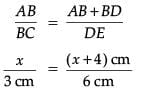6x = 3(x + 4)

6x = 3x + 12

6x – 3x = 12

3x = 12 x = 4

Hence, AB = 4 cm.

Case Based Questions Test: Triangles - 1 - Question 2

### Direction: Read the following text and answer the below questions:SCALE FACTORA scale drawing of an object is the same shape at the object but a different size. The scale of a drawing is a comparison of the length used on a drawing to the length it represents. The scale is written as a ratio. The ratio of two corresponding sides in similar figures is called the scale factorScale factor = length in image / corresponding length in objectIf one shape can become another using revising, then the shapes are similar. Hence, two shapes are similar when one can become the other after a resize, flip, slide or turn. In the photograph below showing the side view of a train engine. Scale factor is 1:200This means that a length of 1 cm on the photograph above corresponds to a length of 200 cm or 2 m, of the actual engine. The scale can also be written as the ratio of two lengths.What will affect the similarity of any two polygons?

Detailed Solution for Case Based Questions Test: Triangles - 1 - Question 2
The common way of defining the Similarity of polygons is

Any two polygons are said to be similar if they are of the same shape and they can be of different sizes.

The Mathematical definition is, Any two polygons are said to be similar if they satisfy the below two conditions.

• The corresponding sides of both the polygons are in the same proportion.

• The corresponding interior angles of the polygons are the same.

Case Based Questions Test: Triangles - 1 - Question 3

### Direction: Read the following text and answer the below questions:SCALE FACTORA scale drawing of an object is the same shape at the object but a different size. The scale of a drawing is a comparison of the length used on a drawing to the length it represents. The scale is written as a ratio. The ratio of two corresponding sides in similar figures is called the scale factorScale factor = length in image / corresponding length in objectIf one shape can become another using revising, then the shapes are similar. Hence, two shapes are similar when one can become the other after a resize, flip, slide or turn. In the photograph below showing the side view of a train engine. Scale factor is 1:200This means that a length of 1 cm on the photograph above corresponds to a length of 200 cm or 2 m, of the actual engine. The scale can also be written as the ratio of two lengths.If two similar triangles have a scale factor 5:3 which statement regarding the two triangles is true?

Detailed Solution for Case Based Questions Test: Triangles - 1 - Question 3
25:15= 25/15 = 5/3.

As 5 divides both. ( i. e., 5 x 5 = 25, 5 x 3 = 15)

Case Based Questions Test: Triangles - 1 - Question 4

SCALE FACTOR

A scale drawing of an object is the same shape at the object but a different size. The scale of a drawing is a comparison of the length used on a drawing to the length it represents. The scale is written as a ratio. The ratio of two corresponding sides in similar figures is called the scale factor

Scale factor = length in image / corresponding length in objectIf one shape can become another using revising, then the shapes are similar. Hence, two shapes are similar when one can become the other after a resize, flip, slide or turn. In the photograph below showing the side view of a train engine. Scale factor is 1:200

This means that a length of 1 cm on the photograph above corresponds to a length of 200 cm or 2 m, of the actual engine. The scale can also be written as the ratio of two lengths.

If the length of the model is 11 cm, then the overall length of the engine in the photograph above, including the couplings(mechanism used to connect) is:

Detailed Solution for Case Based Questions Test: Triangles - 1 - Question 4

Given that

Scale factor is 1:200

Therefore,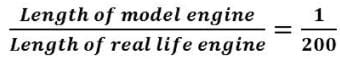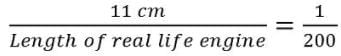11 x 200 cm = Length of real life engine

2200 cm = Length of real life engine

Length of real life engine = 2200 cm

Length of real life engine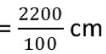Length of real life engine = 22 m.

Case Based Questions Test: Triangles - 1 - Question 5

SCALE FACTOR

A scale drawing of an object is the same shape at the object but a different size. The scale of a drawing is a comparison of the length used on a drawing to the length it represents. The scale is written as a ratio. The ratio of two corresponding sides in similar figures is called the scale factor

Scale factor = length in image / corresponding length in objectIf one shape can become another using revising, then the shapes are similar. Hence, two shapes are similar when one can become the other after a resize, flip, slide or turn. In the photograph below showing the side view of a train engine. Scale factor is 1:200

This means that a length of 1 cm on the photograph above corresponds to a length of 200 cm or 2 m, of the actual engine. The scale can also be written as the ratio of two lengths.

What is the actual width of the door if the width of the door in photograph is 0.35 cm?

Detailed Solution for Case Based Questions Test: Triangles - 1 - Question 5
Given that

Scale factor is 1:200

Therefore,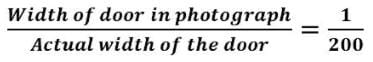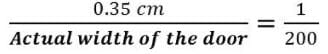0.35 x 200 cm = Actual width of the door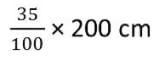= Actual width of the door

35 x 2 cm = Actual width of the door

70 cm = Actual width of the door

Actual width of the door = 70 cm

Actual width of the door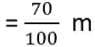Actual width of the door = 0.7 m.

Case Based Questions Test: Triangles - 1 - Question 6

Seema placed a light bulb at point O on the ceiling and directly below it placed a table. Now, she put a cardboard of shape ABCD between table and lighted bulb. Then a shadow of ABCD is casted on the table as A'B'C'D' (see figure). Quadrilateral A'B'C'D' in an enlargement of ABCD with scale factor 1 : 2, Also, AB = 1.5 cm, BC = 25 cm, CD = 2.4 cm and AD = 2.1 cm; ∠A = 105°, ∠B = 100°, ∠C = 70° and ∠D = 85°.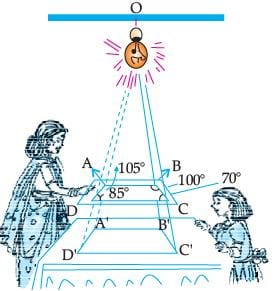What is the sum of angles C' and D'?

Detailed Solution for Case Based Questions Test: Triangles - 1 - Question 6
∠C' = ∠C = 70°

and ∠D' = ∠D = 85°

∴ ∠C' + ∠D' = 70° + 85° = 155°

Case Based Questions Test: Triangles - 1 - Question 7

Seema placed a light bulb at point O on the ceiling and directly below it placed a table. Now, she put a cardboard of shape ABCD between table and lighted bulb. Then a shadow of ABCD is casted on the table as A'B'C'D' (see figure). Quadrilateral A'B'C'D' in an enlargement of ABCD with scale factor 1 : 2, Also, AB = 1.5 cm, BC = 25 cm, CD = 2.4 cm and AD = 2.1 cm; ∠A = 105°, ∠B = 100°, ∠C = 70° and ∠D = 85°.What is the length of A'B' ?

Detailed Solution for Case Based Questions Test: Triangles - 1 - Question 7
Given scale factor is 1 : 2

∴ A'B' = 2AB

⇒ A'B' = 2 × 1.5 = 3 cm

Case Based Questions Test: Triangles - 1 - Question 8

Seema placed a light bulb at point O on the ceiling and directly below it placed a table. Now, she put a cardboard of shape ABCD between table and lighted bulb. Then a shadow of ABCD is casted on the table as A'B'C'D' (see figure). Quadrilateral A'B'C'D' in an enlargement of ABCD with scale factor 1 : 2, Also, AB = 1.5 cm, BC = 25 cm, CD = 2.4 cm and AD = 2.1 cm; ∠A = 105°, ∠B = 100°, ∠C = 70° and ∠D = 85°.What is the ratio of sides A'B' and A'D' ?

Detailed Solution for Case Based Questions Test: Triangles - 1 - Question 8
A'B' = 3 cm

= 2 × 2.1 = 4.2 cm

∴ A'B'/A'D' = 3/4.2 = 30/42

= 5/7 or 5 : 7

Case Based Questions Test: Triangles - 1 - Question 9

Seema placed a light bulb at point O on the ceiling and directly below it placed a table. Now, she put a cardboard of shape ABCD between table and lighted bulb. Then a shadow of ABCD is casted on the table as A'B'C'D' (see figure). Quadrilateral A'B'C'D' in an enlargement of ABCD with scale factor 1 : 2, Also, AB = 1.5 cm, BC = 25 cm, CD = 2.4 cm and AD = 2.1 cm; ∠A = 105°, ∠B = 100°, ∠C = 70° and ∠D = 85°.What is the measurement of angle A' ?

Detailed Solution for Case Based Questions Test: Triangles - 1 - Question 9
Quadrilateral A'B'C'D' is similar to ABCD.

∴ ∠A' = ∠A

⇒ ∠A' = 105°

Case Based Questions Test: Triangles - 1 - Question 10

Seema placed a light bulb at point O on the ceiling and directly below it placed a table. Now, she put a cardboard of shape ABCD between table and lighted bulb. Then a shadow of ABCD is casted on the table as A'B'C'D' (see figure). Quadrilateral A'B'C'D' in an enlargement of ABCD with scale factor 1 : 2, Also, AB = 1.5 cm, BC = 25 cm, CD = 2.4 cm and AD = 2.1 cm; ∠A = 105°, ∠B = 100°, ∠C = 70° and ∠D = 85°.What is the sum of angles of quadrilateral A'B'C'D' ?

Detailed Solution for Case Based Questions Test: Triangles - 1 - Question 10
Sum of the angles of quadrilateral A'B'C'D' is 360°
Case Based Questions Test: Triangles - 1 - Question 11

Rohan wants to measure the distance of a pond during the visit to his native. He marks points A and B on the opposite edges of a pond as shown in the figure below.

To find the distance between the points, he makes a right-angled triangle using rope connecting B with another point C are a distance of 12 m, connecting C to point D at a distance of 40 m from point C and the connecting D to the point A which is are a distance of 30 m from D such the ∠ADC = 90°.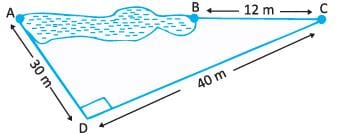Find the length of the rope used.

Detailed Solution for Case Based Questions Test: Triangles - 1 - Question 11
Length of Rope = BC + CD + DA

= 12 m + 40 m + 30 m

= 82 m

Case Based Questions Test: Triangles - 1 - Question 12

Rohan wants to measure the distance of a pond during the visit to his native. He marks points A and B on the opposite edges of a pond as shown in the figure below.

To find the distance between the points, he makes a right-angled triangle using rope connecting B with another point C are a distance of 12 m, connecting C to point D at a distance of 40 m from point C and the connecting D to the point A which is are a distance of 30 m from D such the ∠ADC = 90°.What is the distance AC?

Detailed Solution for Case Based Questions Test: Triangles - 1 - Question 12
According to the pythagoras,

AC2 = (30 m)2 + (40 m)2

AC2 = 900 + 1600

AC2 = 2500

AC = 50 m

Case Based Questions Test: Triangles - 1 - Question 13

Rohan wants to measure the distance of a pond during the visit to his native. He marks points A and B on the opposite edges of a pond as shown in the figure below.

To find the distance between the points, he makes a right-angled triangle using rope connecting B with another point C are a distance of 12 m, connecting C to point D at a distance of 40 m from point C and the connecting D to the point A which is are a distance of 30 m from D such the ∠ADC = 90°.Find the length AB?

Detailed Solution for Case Based Questions Test: Triangles - 1 - Question 13
AC = 50 m

BC = 12 m

AC = AB + BC

50 m = AB + 12 m

AB = 50 m – 12 m

AB = 38 m

Case Based Questions Test: Triangles - 1 - Question 14

Rohan wants to measure the distance of a pond during the visit to his native. He marks points A and B on the opposite edges of a pond as shown in the figure below.

To find the distance between the points, he makes a right-angled triangle using rope connecting B with another point C are a distance of 12 m, connecting C to point D at a distance of 40 m from point C and the connecting D to the point A which is are a distance of 30 m from D such the ∠ADC = 90°.Which property of geometry will be used to find the distance AC?

Detailed Solution for Case Based Questions Test: Triangles - 1 - Question 14

We know two sides AD, CD

And, we find AC using Pythagoras Theorem.

Case Based Questions Test: Triangles - 1 - Question 15

Rohan wants to measure the distance of a pond during the visit to his native. He marks points A and B on the opposite edges of a pond as shown in the figure below.

To find the distance between the points, he makes a right-angled triangle using rope connecting B with another point C are a distance of 12 m, connecting C to point D at a distance of 40 m from point C and the connecting D to the point A which is are a distance of 30 m from D such the ∠ADC = 90°.Which is the following does not form a Pythagoras triplet?

Detailed Solution for Case Based Questions Test: Triangles - 1 - Question 15
We need to check each and every option

Option A - (7, 24, 25)

72 + 242 = 49 + 576

= 625

= 252

So, this is a Pythagorean triplet

Option B - (15, 8, 17)

152 + 82 = 225 + 64

= 289

= 172

So, this is a Pythagorean triplet

Option C - (5, 12, 13)

52 + 122 = 25 + 144

= 169

= 132

So, this is a Pythagorean triplet

Option D - (21, 20, 28)

212 + 202 = 441 + 400

= 881

282

## Mathematics (Maths) Class 10

115 videos|478 docs|129 tests
Information about Case Based Questions Test: Triangles - 1 Page
In this test you can find the Exam questions for Case Based Questions Test: Triangles - 1 solved & explained in the simplest way possible. Besides giving Questions and answers for Case Based Questions Test: Triangles - 1, EduRev gives you an ample number of Online tests for practice

## Mathematics (Maths) Class 10

115 videos|478 docs|129 tests